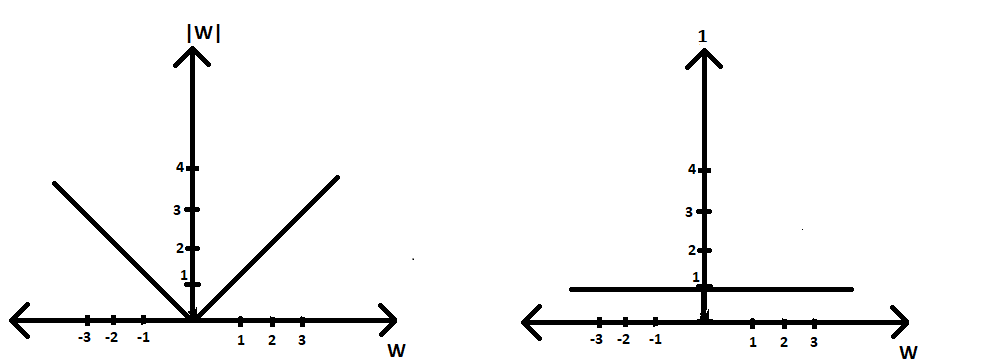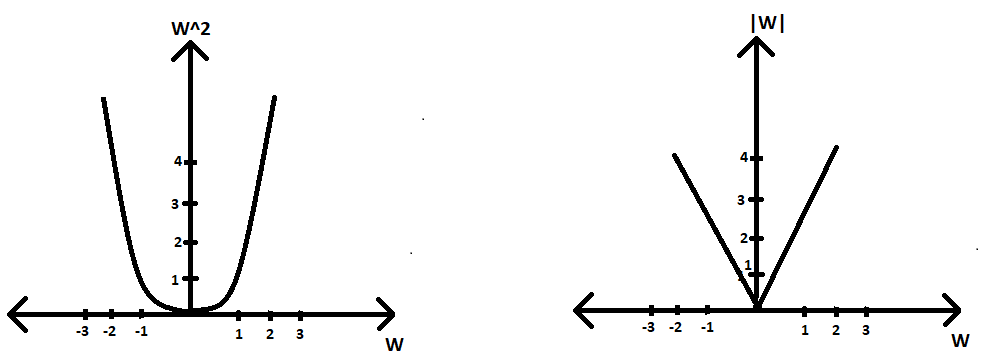# How L1 Regularization brings Sparsity

• Last Updated : 09 Jun, 2021

Prerequisites: Regularization in ML

We know that we use regularization to avoid underfitting and over fitting while training our Machine Learning models. And for this purpose, we mainly use two types of methods namely: L1 regularization and L2 regularization.

Attention reader! Don’t stop learning now. Get hold of all the important Machine Learning Concepts with the Machine Learning Foundation Course at a student-friendly price and become industry ready.

L1 regularizer :

||w||1=( |w1|+|w2|+ . . . + |wn

L2 regularizer :

||w||2=( |w1|2+|w2|2+ . . . + |wn|2 )1/2

(where w1,w2 … wn are ‘d‘ dimensional weight vectors)

Now while optimization, that is done based on the concept of Gradient Descent algorithm, it is seen that if we use L1 regularization, it brings sparsity to our weight vector by making smaller weights as zero. Let’s see how but first let’s understand some basic concepts

Sparse vector or matrix: A vector or matrix with a maximum of its elements as zero is called a sparse vector or matrix.Wt=W(t-1) – η* ( ∂L(w)/∂(w) )W(t-1)

(where η is a small value called learning rate)

As per the gradient descent algorithm, we get our answer when convergence occurs. Convergence occurs when the value of Wt doesn’t change much with further iterations, or we can say when we get minima i.e. ( ∂(Loss)/∂w )W(t-1) becomes approximately equal to 0 an thus, Wt ~ Wt-1.

Let’s assume we have a linear regression optimization problem(we can take that for any model), with its equation as:

argmin(W) [ loss + (regularizer) ]
i.e. Find that W which will minimize the loss function

Here we will ignore the loss term and only focus on the regularization term for making it easier for us to analyze our task.

Case1 (L1 taken) :

Optimisation equation= argmin(W) |W|

(∂|W|/∂w) = 1

thus, according to GD algorithm Wt = Wt-1 - η*1

Here as we can see our loss derivative comes to be constant, so the condition of convergence occurs faster because we have only η in our subtraction term and it is not being multiplied by any smaller value of W. Therefore our Wt tends towards 0 in a few iterations only. But this will get hinder in our condition to convergence to occur as we will see in the next case.Plot of L1 regularizer vs w(∂|W|/ ∂w) vs w

Case2 (L2 taken) :

Optimisation equation=argmin(W) |W|2

(∂|W|2/∂W) = 2|W|

thus, according to GD algorithm Wt = Wt-1 - 2*η*|W|`

Hence, we can see that our loss derivative term is not constant and thus for smaller values of W, our condition of convergence will not occur faster(or maybe at all) because we have a smaller value of W getting multiplied with η and thus making the whole term to be subtracted even smaller. Therefore, after a few iterations, our Wt becomes a very small constant value but not zero. Hence, not contributing to the sparsity of the weight vector.Plot of L2 regularizer vs w ; Right Side: Plot of  ∂|W|2/∂W) vs w

My Personal Notes arrow_drop_up